Test: Analog Communication - 2

# Test: Analog Communication - 2 - Electronics and Communication Engineering (ECE)

Test Description

## 20 Questions MCQ Test GATE ECE (Electronics) 2023 Mock Test Series - Test: Analog Communication - 2

Test: Analog Communication - 2 for Electronics and Communication Engineering (ECE) 2023 is part of GATE ECE (Electronics) 2023 Mock Test Series preparation. The Test: Analog Communication - 2 questions and answers have been prepared according to the Electronics and Communication Engineering (ECE) exam syllabus.The Test: Analog Communication - 2 MCQs are made for Electronics and Communication Engineering (ECE) 2023 Exam. Find important definitions, questions, notes, meanings, examples, exercises, MCQs and online tests for Test: Analog Communication - 2 below.
Solutions of Test: Analog Communication - 2 questions in English are available as part of our GATE ECE (Electronics) 2023 Mock Test Series for Electronics and Communication Engineering (ECE) & Test: Analog Communication - 2 solutions in Hindi for GATE ECE (Electronics) 2023 Mock Test Series course. Download more important topics, notes, lectures and mock test series for Electronics and Communication Engineering (ECE) Exam by signing up for free. Attempt Test: Analog Communication - 2 | 20 questions in 60 minutes | Mock test for Electronics and Communication Engineering (ECE) preparation | Free important questions MCQ to study GATE ECE (Electronics) 2023 Mock Test Series for Electronics and Communication Engineering (ECE) Exam | Download free PDF with solutions
 1 Crore+ students have signed up on EduRev. Have you?
*Answer can only contain numeric values
Test: Analog Communication - 2 - Question 1

### An AM signal is represented in the domain as S (-c) = 4 cos 1800T + 10 cos 20002 + 4cos2200T then the bandwidth of the AM signal is _____ Hz

Test: Analog Communication - 2 - Question 2

### A 100MHz carrier is frequency modulated by 10 KHz wave. For a frequency deviation of 50 KHz, calculate the modulation index of the FM signal.

Detailed Solution for Test: Analog Communication - 2 - Question 2

Carrier frequency fc = 100MHz
Modulating frequency fm = 10 KHz
Frequency deviation Δf = 500 KHz
Modulation index of FM signal is given by
mf = Δf/fm
= 500 * 103/ 10 * 103
= 50

Test: Analog Communication - 2 - Question 3

### Consider a system shown in the figure-1. Let X(f) and Y(f) represent the Fourier transforms of x(t) and y(t) respectively. The ideal HPF has cutoff frequency of 10 KHZ. X(f) is shown in the figure-2. The positive frequency where Y(f) has spectral peaks are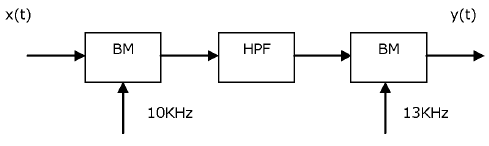​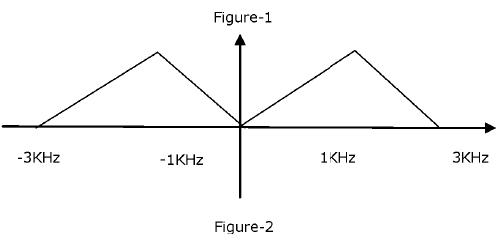Detailed Solution for Test: Analog Communication - 2 - Question 3

2 KHz S. 24 KHz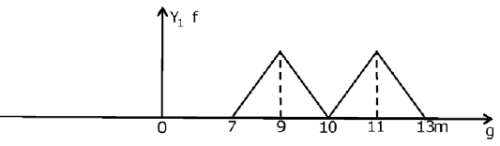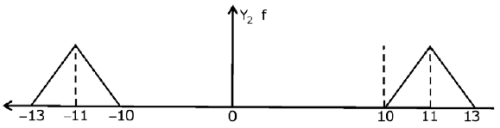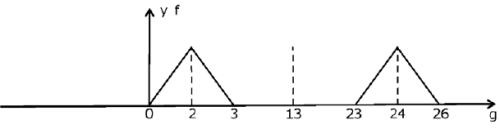Test: Analog Communication - 2 - Question 4

Three message signals each band limited to 10 kHz, 10 kHz, and 5 KHz are transmitted through a channel using frequency division multiplexing. The modulation techniques used are any, D513-SC, SSB respectively. The guard band is 2 KHz. Band width of the channel is

Detailed Solution for Test: Analog Communication - 2 - Question 4

BW after AM modulation = 2 x 10 = 20 KHz

BW after DSS-SC modulation = 2 x10 = 20 KHz BW after SSB modulation = 5 KHz

Guard band width = 2 x 2k=4 k

∴ Total BW after frequency multiplexing = 49 KHz

Test: Analog Communication - 2 - Question 5

A signal '4 t is band limited to the frequency range 0 to fn. The signal frequency is translated by multiplying it by the signal V, t —cos2πfct . The value of fc such that the bandwidth of the translated signal is 1% of the carrier frequency fc will be

Detailed Solution for Test: Analog Communication - 2 - Question 5

BW after multiplexing =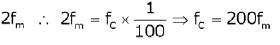*Answer can only contain numeric values
Test: Analog Communication - 2 - Question 6

The peak amplitude of an AM signal for tone modulation with carrier having maximum amplitude as 5 V, varies from 2 to 8 V then the sideband power is  _________________________ (W)

Detailed Solution for Test: Analog Communication - 2 - Question 6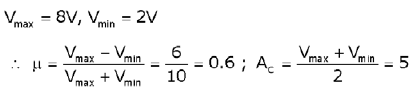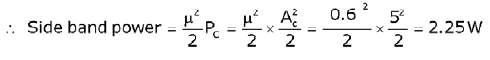Test: Analog Communication - 2 - Question 7

Consider a frequency multiplier shown in the figure below.ss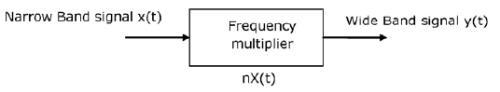A NBFM signalX Mal t with f, = 200KHz and f, ranges 50 Hz to 150 KHz is given as an input to frequency multiplier. The Maximum Frequency deviation of the NBFM is 25 Hz. The maximum frequency deviation at the output is 75 KHz.

Q. The required frequency multiplication n is

Detailed Solution for Test: Analog Communication - 2 - Question 7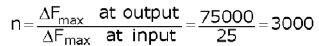Test: Analog Communication - 2 - Question 8

Consider a frequency multiplier shown in the figure below.ssA NBFM signalX Mal t with f, = 200KHz and f, ranges 50 Hz to 150 KHz is given as an input to frequency multiplier. The Maximum Frequency deviation of the NBFM is 25 Hz. The maximum frequency deviation at the output is 75 KHz.

Q. The carrier frequency at the output is

Test: Analog Communication - 2 - Question 9

SSB modulation may be viewed as a hybrid form of amplitude modulation and frequency modulation. Assuming a message signal m(t) = sin t and a carrier frequency 'fc', the instantaneous frequency for the lower side band is

Detailed Solution for Test: Analog Communication - 2 - Question 9

For SSB modulation, the modulated wave is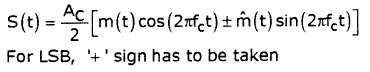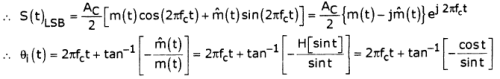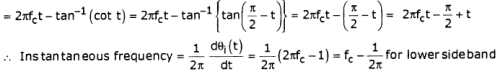Test: Analog Communication - 2 - Question 10

Given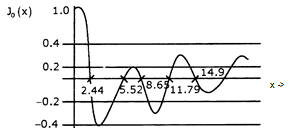In a certain experimental conducted with fm = 1 kHz and increasing Am(starting from 0 volts), it is found that the carrier component of the FM signal is reduced to zero for the first time when Am = 2 volts.

The frequency sensitivity of the modulator is

Detailed Solution for Test: Analog Communication - 2 - Question 10

When Am = 2V, carrier component = 0

ie. J0 (03) = 0 for the 1st time

From the curve, corresponding (3=2.44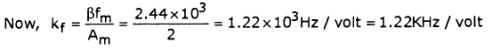*Answer can only contain numeric values
Test: Analog Communication - 2 - Question 11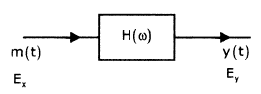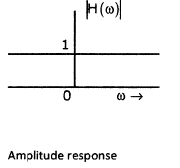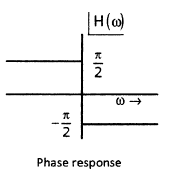If Ex and Ey represent the energies of m(t) and y(t) respectively, then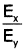_______________

Detailed Solution for Test: Analog Communication - 2 - Question 11

Mh (ω) = -jM (6)) sgn(ω), (The given system is a Hilbert transformer) The π of a Hilbert transformer is

H (ω) = -jsgn (ω)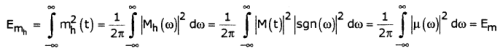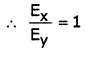Test: Analog Communication - 2 - Question 12

Determine the spectrum of y(t) in the system shown below.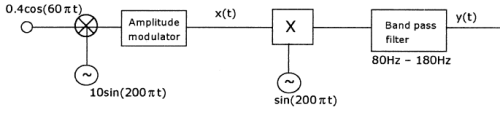Detailed Solution for Test: Analog Communication - 2 - Question 12

Output of AM Modulator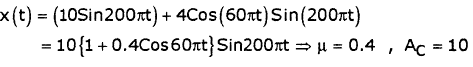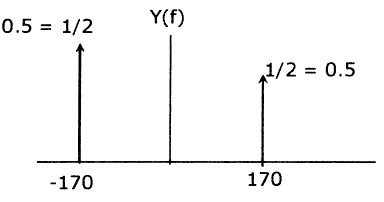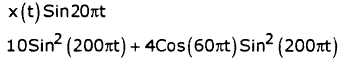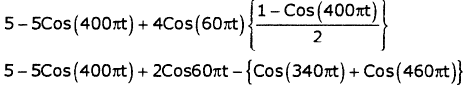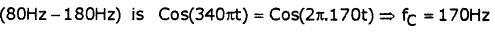Test: Analog Communication - 2 - Question 13

Consider the scheme shown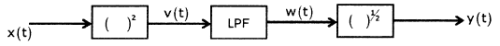If x (t) = m (t) cos (coct) then y (t) is

Detailed Solution for Test: Analog Communication - 2 - Question 13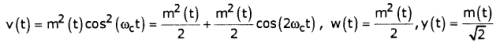Test: Analog Communication - 2 - Question 14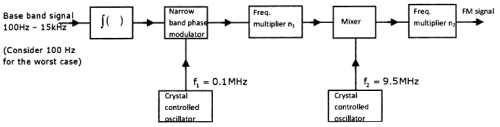The above schematic generates FM signal as a wideband frequency modulator

using the indirect method. The output FM signal is characterized by fc = 100 MHz, Δf = 75kHz (min). If the phase modulator has a modulation index of β= 0.3 radians, then n1, n2 are respectively

Detailed Solution for Test: Analog Communication - 2 - Question 14

The lowest modulation frequency i.e.,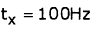Produces a frequency deviation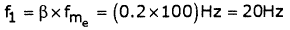The highest modulation frequency ie.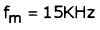Produces a frequency deviation of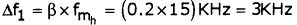Let us take the case of 100Hz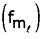as the worst case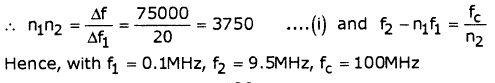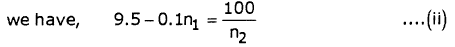Solving (1) & (2) we get,

n1=75 , n2 =50

Test: Analog Communication - 2 - Question 15

What is the standard IF frequency for AM receivers?

Detailed Solution for Test: Analog Communication - 2 - Question 15

In AM receivers, the standard intermediate frequency is approximately 455 KHz.

Test: Analog Communication - 2 - Question 16

Consider a message signal m(t) = 2sin(10007t)

Frequency sensitivity constant Kf=75KHz/V

Q. If amplitude of message signal is doubled, then bandwidth for FM and PM will be

Detailed Solution for Test: Analog Communication - 2 - Question 16

Δf = 75x 4 = 300KHz ,

BFM = 2(300 + 0.5) = 601KHz, Δ f= 2 x Kp x 2 x 500 = 10KHz,Bpm = 2(10 + 0.5) = 21KHz

Test: Analog Communication - 2 - Question 17

A 20 KW and 1 MHz carrier is frequency modulated by a sinusoidal signal so that the maximum frequency deviation is 50 KHz and the message signal is band limited to 500 KHz. The generated FM signal is amplitude modulated using carrier of 1 GHz.

Q. The bandwidth of the generated signal is

Detailed Solution for Test: Analog Communication - 2 - Question 17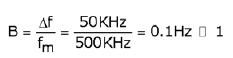It is a narrow band FM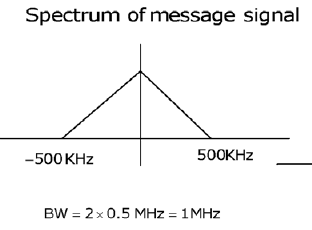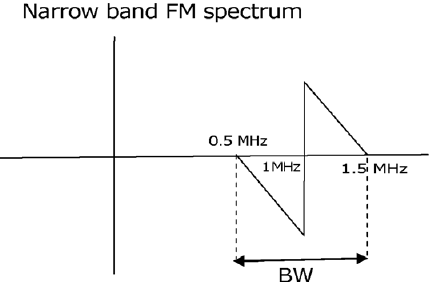Test: Analog Communication - 2 - Question 18

A 20 KW and 1 MHz carrier is frequency modulated by a sinusoidal signal so that the maximum frequency deviation is 50 KHz and the message signal is band limited to 500 KHz. The generated FM signal is amplitude modulated using carrier of 1 GHz.

Q. The bandwidth of the generated AM signal is

Detailed Solution for Test: Analog Communication - 2 - Question 18

Taking above spectrum as message signal and generate AM signal using carrier 1GHZ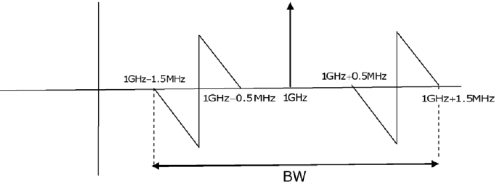BW = 2 1,5 MHz = 3MHz

Test: Analog Communication - 2 - Question 19

The input x(t) and the output y(t) of a certain nonlinear channel are related as y(t) = x(t) + 0.001 x2(t)

If frequency spectrum of input signal is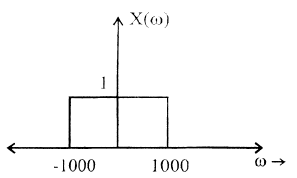Q. The bandwidth of the output signal is

Test: Analog Communication - 2 - Question 20

The input x(t) and the output y(t) of a certain nonlinear channel are related as y(t) = x(t) + 0.001 x2(t)

If frequency spectrum of input signal isQ. Spectrum of the received signal after appropriate low pass filtering is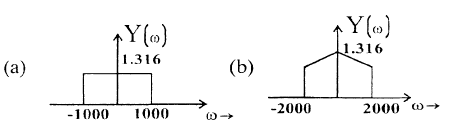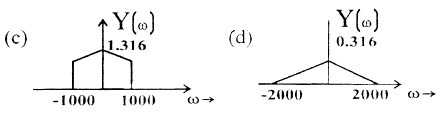## GATE ECE (Electronics) 2023 Mock Test Series

21 docs|263 tests
Information about Test: Analog Communication - 2 Page
In this test you can find the Exam questions for Test: Analog Communication - 2 solved & explained in the simplest way possible. Besides giving Questions and answers for Test: Analog Communication - 2, EduRev gives you an ample number of Online tests for practice

## GATE ECE (Electronics) 2023 Mock Test Series

21 docs|263 tests(Scan QR code)